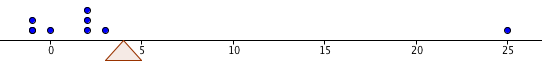﻿ Mean, Median, and Mode
MEAN, MEDIAN, and MODE

Statistics is the discipline devoted to organizing, summarizing, and drawing conclusions from data.

Given a collection of data, it is often convenient to come up with a single number that somehow describes its center;
a number that in some way is representative of the entire collection.
Such a number is called a measure of central tendency.

The two most popular measures of central tendency are the mean and the median.
Another measure sometimes used to describe a ‘typical’ data value is the mode.

the MEAN of a data set

The mean (or average) is already familiar to you: add up the numbers, and divide by how many there are:

DEFINITION mean, average
The mean (or average) of the $\,n\,$ data values $$\,x_1, x_2, x_3, \ldots, x_n\,$$ is denoted by $\,\bar{x}\,$ (read as ‘$\,x\,$ bar’) and is given by the formula \begin{alignat}{2} \bar{x}\ &= \frac{x_1 + x_2 + x_3 + \cdots + x_n}{n}\\ &= \frac{\sum_{i=1}^n\ x_i}{n}\ \ &&\text{(using summation notation)}\\ &= \frac1n\sum_{i=1}^n\ x_i\ \ &&\text{(an alternate version of summation notation)}\\ \end{alignat} Thus, to find the mean of $\,n\,$ data values, you add them up and then divide by $\,n\,$.

Similarly, the mean of the $\,n\,$ data values   $\,y_1, y_2, y_3, \ldots, y_n\,$
would be denoted by $\,\bar{y}\,$ and read as ‘$\,y\,$ bar’.

Since dividing by $\,n\,$ is the same as multiplying by $\,\frac{1}{n}\,$,
the notation $\displaystyle\,\frac{\sum_{i=1}^n\ x_i}{n}\,$ is more commonly written as   $\,\frac 1n\sum_{i=1}^n\ x_i\,$   or   $\displaystyle\,\frac 1n\sum_{i=1}^n\ x_i\,$  .

EXAMPLE:
Find the mean of these data values:   $2,\ -1,\ 2,\ 3,\ 0,\ 25,\ -1,\ 2$
There are $\,8\,$ data values.
The mean is found by adding them up and then dividing by $\,8\,$: $$\frac{2+(-1)+2+3+0+25+(-1)+2}{8} = \frac{32}{8} = 4$$ As discussed in Average of Three Signed Numbers,
the mean gives the balancing point for the distribution, in the following sense:
if eight pebbles of equal weight are placed on a ‘number line see-saw’:
two pebbles at $\,-1\,$, one pebble at $\,0\,$, three pebbles at $\,2\,$, one pebble at $\,3\,$, and one pebble at $\,25\,$;
then the support would have to be placed at $\,4\,$ for the see-saw to balance perfectly!Notice in the previous example that the number $\,25\,$ seems to be unusually large, compared to the other numbers.
An outlier is an unusually large or small observation in a data set.
A drawback of the mean is that its value can be greatly affected by the presence of even a single outlier.
If the outlier $\,25\,$ is changed to $\,250\,$, then the new mean would be $\,32.125\,$,
which does not seem at all representative of a ‘typical’ number in this data set!

the MEDIAN of a data set

The median, on the other hand, is quite insensitive to outliers.

Just as the median strip of a highway goes right down the middle,
the median of a set of numbers goes right through the middle of the ordered list.
Of course, only lists with an odd number of values have a true middle:
the middle number in the ordered list $\,5,\ 7,\ 20\,$ is $\,7\,$.
See how the definition below solves the problem when there are an even number of data values:

DEFINITION median
To find the median of a set of $\,n\,$ data values,
first order the observations from least to greatest (or greatest to least).

If $\,n\,$ is odd, then the median is the number in the exact middle of the list.
That is, the median is the data value in position $\,\frac{n+1}{2}\,$ of the ordered list.

If $\,n\,$ is even, then the median is the average of the two middle members of the ordered list.
That is, the median is the average of the data values in positions $\,\frac{n}{2}\,$ and $\,\frac{n}{2}+1\,$
of the ordered list.
EXAMPLE:
Question:
Find the median of these data values:   $\,2,\ -1,\ 2,\ 3,\ 0,\ 25,\ -1,\ 2$
(This is the same data set as in the previous example.)
Solution:
Begin by ordering the eight data values from least to greatest: $$\underset{\text{position 1}}{\underset{\uparrow}{-1,\strut}}\ \ \ \ \underset{\text{position 2}}{\underset{\uparrow}{-1,\strut}}\ \ \ \ \underset{\text{position 3}}{\underset{\uparrow}{0,\strut}}\ \ \ \ \overset{\text{the two ‘middle’ members}} {\ \ \overbrace{ \underset{\text{position 4}}{\underset{\uparrow}{2,\strut}}\ \ \ \ \underset{\text{position 5}}{\underset{\uparrow}{2,\strut}} }}\ \ \ \ \underset{\text{position 6}}{\underset{\uparrow}{2,\strut}}\ \ \ \ \underset{\text{position 7}}{\underset{\uparrow}{3,\strut}}\ \ \ \ \underset{\text{position 8}}{\underset{\uparrow}{25\strut}}$$ There are an even number of values, so we average the values in positions four and five:
the median is $\,\frac{2+2}{2} = 2\,$.

Note that, for this data set, the median seems to do a better job than the mean in representing a ‘typical’ member.
Note also that if the outlier $\,25\,$ is changed to $\,250\,$, it doesn't affect the median at all!
the MODE of a data set

Finally, a mode is a value that occurs ‘most often’ in a data set.
Whereas a data set has exactly one mean and median, it can have one or more modes.

For example, consider these data values:   $\,2,\ -1,\ 2,\ 3,\ 0,\ 25,\ -1,\ 2\,$
Re-group them occurring to their frequency: \begin{align} 2,\ \ 2,\ \ 2,\ \ \ \ &\text{three occurrences of the number 2}\\ -1,\ \ -1,\ \ \ \ &\text{two occurrences of the number -1}\\ 0,\ \ \ \ &\text{one occurrence of the number 0}\\ 3,\ \ \ \ &\text{one occurrence of the number 3}\\ 25\ \ \ \ &\text{one occurrence of the number 25} \end{align} The mode of this data set is $\,2\,$, since this data value occurs three times, and this is the most occurrences of any data value.

Every member of the data set   $\,3,\ 7,\ 9\,$   is a mode, since each value occurs only once.

The data set   $\,3,\ 3,\ 7,\ 7,\ 9\,$   has two modes: $\,3\,$ and $\,7\,$.
Each of these numbers occurs twice, and no number occurs more than two times.

Master the ideas from this section